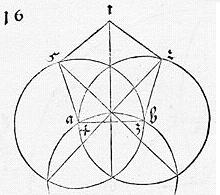## Approximate Construction of Regular Pentagon by A. Dürer

Albrecht Dürer (1471-1528), a pioneer German painter, engraver and a famous amateur mathematician, was known to be interested, among many other things, in pentagonal plane tessellations, pentagonal ornaments and practical construction of regular polygons, pentagons in particular. In his 1525 book, Underweysung der Messung, mit dem Zirckel und Richtscheyt (Treatise on Mensuration with Compass and Ruler), intended for architects and draftsmen he gave an approximate construction of a regular pentagon. The construction uses straightedge and compass whose opening is set only once and remains fixed in the course of the construction.1. Start with a segment AB and draw two circles A(B) and B(A), one with center A and passing through B, the other with center B and passing through A. Mark the intersection points N and Q of the two circles.

2. With the center Q and the same radius draw circle Q(A) passing through A and B. Mark point P where it crosses line NQ.

3. Let E be the intersection of PS with A(B) and C the intersection of PR with B(A).

4. Mark D the intersection of E(A) and C(B), as shown.

ABCDE is an almost regular pentagon. Let's see that this is indeed so.

Concentrate on the circle centered at Q, (Q). By construction,

 BS = AB = AR = QR = QS = 1,

as if we were trying to inscribe a regular hexagon into (Q). It follows that

1. RS is a diameter of (Q),
2. ∠RAS = ∠RBS = 90°,
3. ∠BRS = 30°,
4. BR = 3/2·RS = 3 (since RS = 2),
5. ∠ARB = 30°,
6. ∠ARP = ∠BRP = 15°.

Apply the Law of Sines to ΔBCR:

 sin(∠BRC) / BC = sin(∠BCR) / BR,

wherefrom

 sin(∠BCR) = √3·sin(15°),

which gives ∠BCR ≈ 26.6338798...°. ∠CBR then is approximately 138.36612...° and, since ∠ABR = 30°, ∠ABC ≈ 108.36612..°, very close to 108° - the internal angle of the regular pentagon. Of course angles ABC and BAE are equal.

We can further determine the remaining angles of ABCDE: angles at C and E are a little less than 108° while the angle at D is a little more than 109° - still not too bad. Here is the original Dürer diagram, as appears in Underweysung der Messung, mit dem Zirckel und Richtscheyt (thanks to C. Reineke for pointing me to the source.)It is a curious observation that Dürer has not mentioned the fact that the construction is merely approximate listing it along with an exact one.

As a matter of fact, the approximate construction is not always credited to Dürer. I received the following note from Dave Blake:

 The construction of an approximate pentagon from a vesica is often credited to Albrecht Dürer, but in fact no one knows for certain where it originally comes from. I thought that you might be interested in some earlier references to this construction that I came across in an addendum to "The Geometer's Angle No. 4: From Pentagon to Heptagon: A Discovery on the Generation of the Regular Heptagon from the Equilateral Triangle and Pentagon" by Mark A Reynolds alias "Marcus the Marinite". The original article was published in NEXUS NETWORK JOURNAL VOL. 3, NO 2, 2001, and having found the addendum online some time ago it no longer seems to be available so I can't give a link. Mark Reynold's points out that the construction is found in the book "Geometrica Deutsch" probably published by Roriczer ca. 1472-1484. So it exists 50 years before the 1525 publication of Underweysung der Messung, mit dem Zirckel und Richtscheyt (Treatise on Mensuration with Compass and Ruler) by Dürer, in which this drawing can be found. It also subsequently appears in "La pratica della perspettiva" by Daniele Barbaro published 1569. His construction of a heptagon is also worth exploring.

### References

1. R. A. Simon, Approximate Construction of Regular Polygons: Two Renaissance Artists, Convergence, MAA
2. D. Wells, The Penguin Dictionary of Curious and Interesting Geometry, Penguin Books, 1991